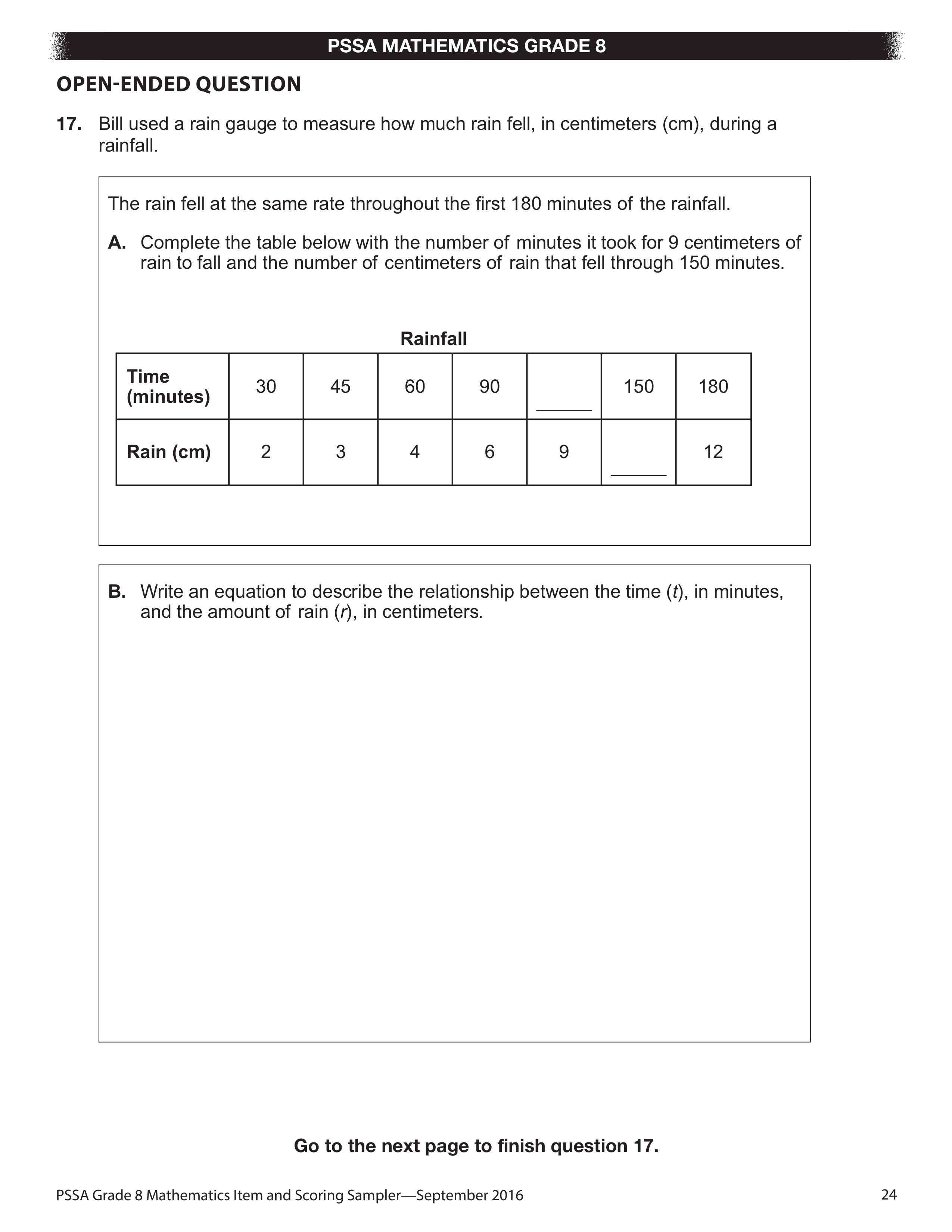(Thursday) Proportional relationships, lines, and linear equations.
starstarstarstarstarstarstarstarstarstar
by Lonnie Myers
| 4 Questions
Note from the author:
Analyze and describe linear relationships between two variables, using slope.1
2
1
1 pt
Complete the table below with the number of minutes it took for 9 centimeters of rain to fall and the number of centimeters of rain that fell through 150 minutes.
2
1 pt
Write an equation to describe the relationship between the time (t), in minutes, and the amount of rain (r), in centimeters.3
4
3
1 pt
Describe the change in the slope between the first 180 minutes and the following 100 minutes, and explain what it means in terms of the total amount of rainfall.

4
1 pt
After 280 minutes, the slope of the graph is 0. Explain what a slope of 0 means in this situation.

Pa Department of Education. 2016 Mathematics ADA Compliant Item and Scoring Sampler Grade 8